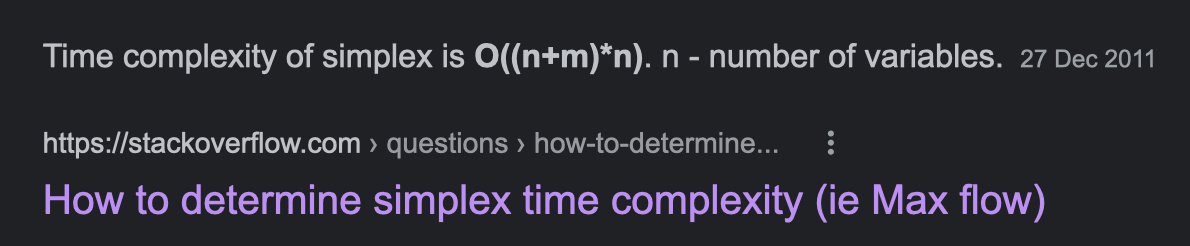# 数据结构与算法： 单纯形法

## Simplex Algorithm and Linear Programming

Posted by R1NG on March 12, 2022 Viewed Times

# 单纯形法

## 单纯形法的引子: 线性规划问题的图像化表示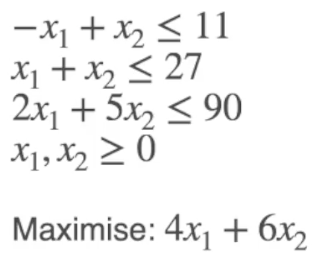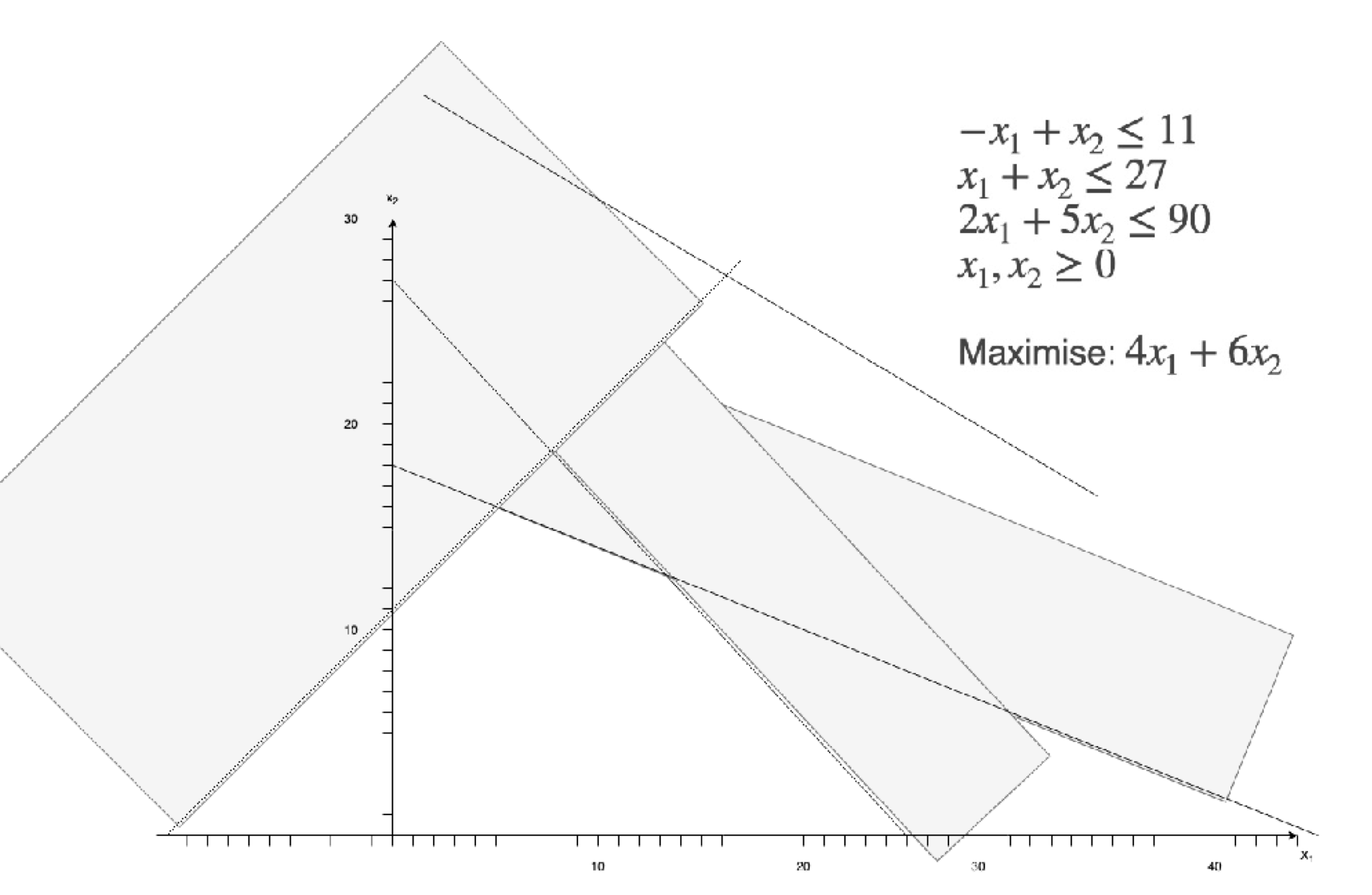## 解决原点为可行解的线性规划问题: 简易单纯形法

### 单纯形法的基石: 简易单纯形法的基本原理

1. 为了确保可行域形为凸超多边形, 单纯形法要求被求解的线性规划问题在矩阵表示形式下满足

$\mathbf{b} \geqslant 0$

也就是说线性规划问题在被转换为标准形式前, 其表示中不会出现 $=$ 或 $\leqslant$.

2. 基于第一点的约束, 不难看出在满足上述条件的问题下 $0$ (零向量, 对应平面的原点) 必为该类问题的一个解.

### 单纯形法中的重要定义: 松弛形式和基础/非基础变量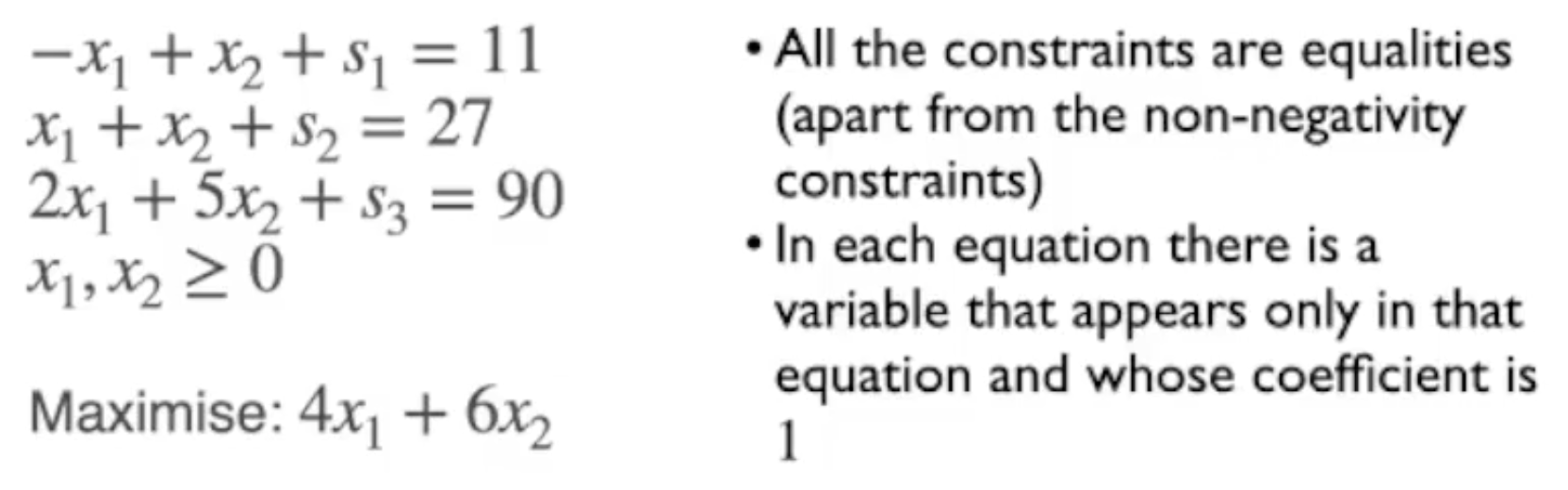1. 对于限制变量为非负的约束规则 (也就是途中从上往下数的第四条规则), 不需要引入任何松弛变量.
2. 对于任何其他的约束规则, 都 只引入一个 约束变量.
3. 任何约束变量的系数 都是 $1$.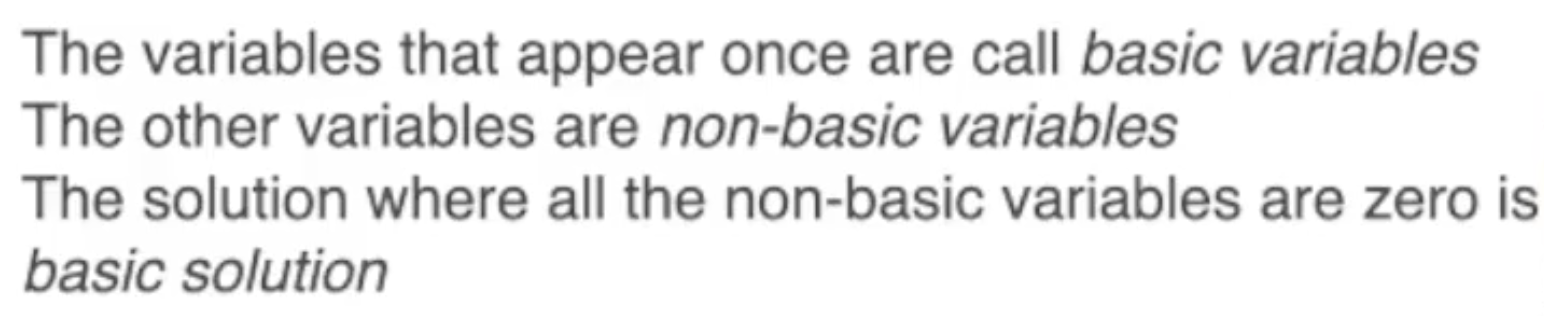### 寻找线性约束问题的最优解: 利用松弛形式 (Slack Form)

1. 所有的松弛变量 都是 基础变量
2. 约束问题中所有的原始变量 都是 非基础变量.

### 在单纯形法中遍历可行解: 枢轴 (Pivoting)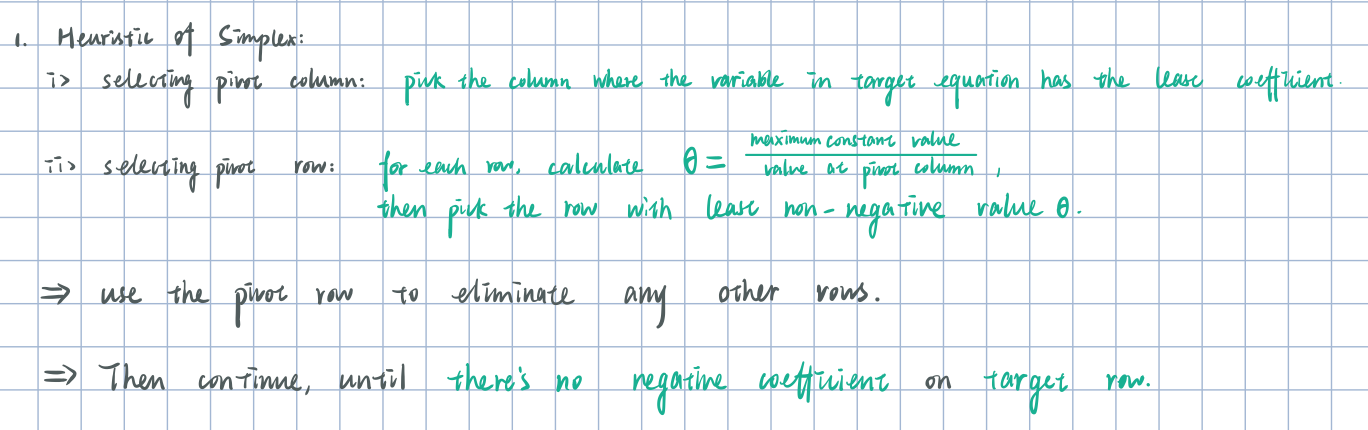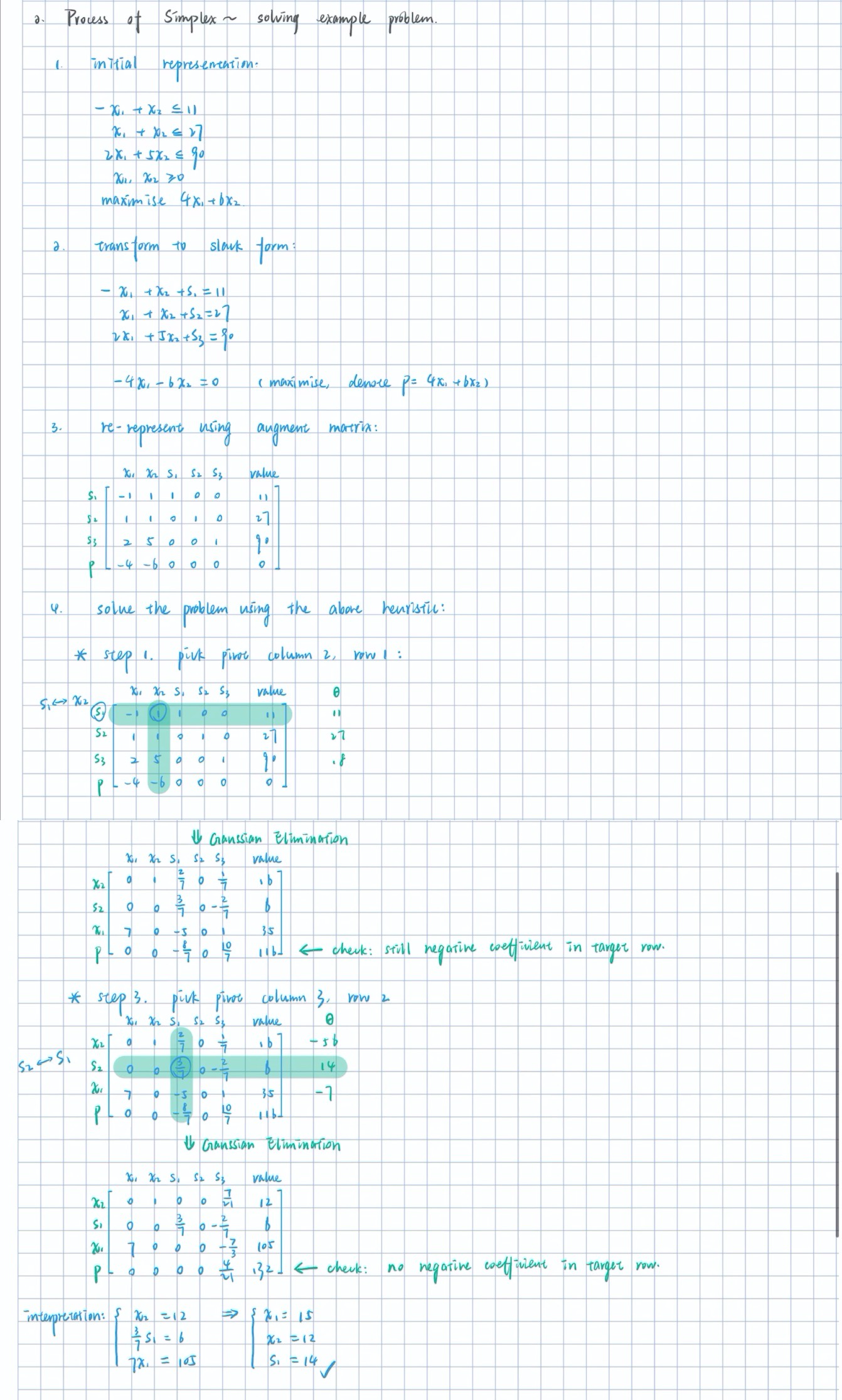## 处理原点不为可行解的线性规划问题: 进阶单纯形法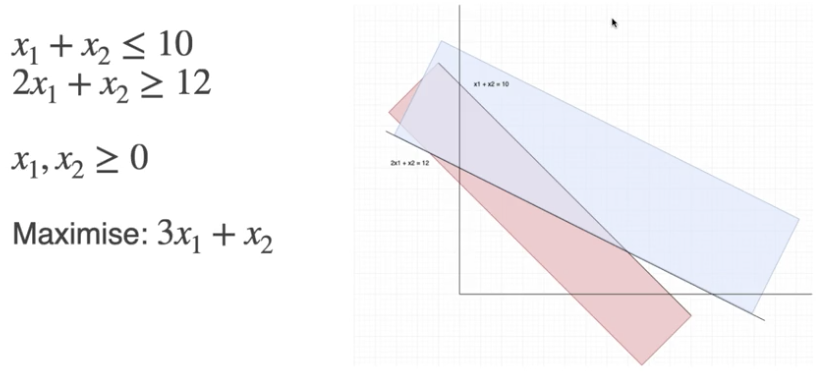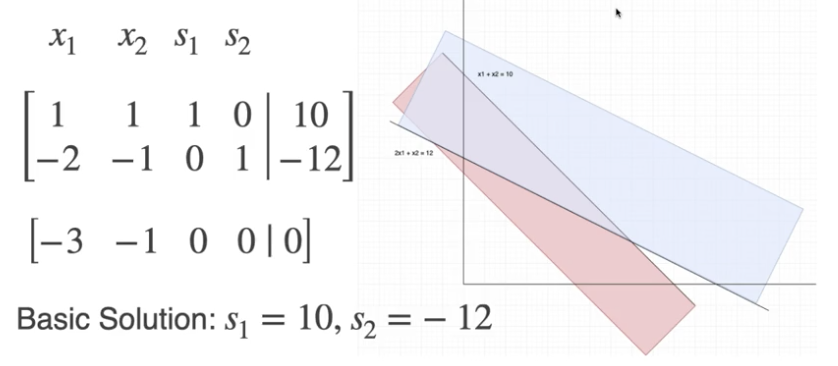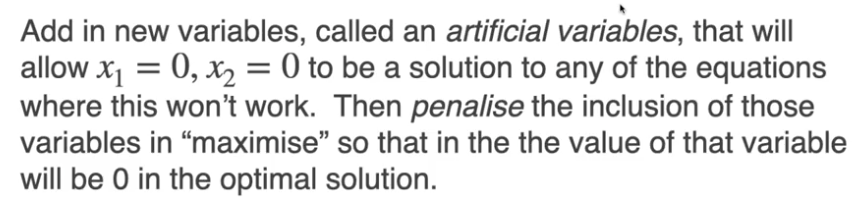1. 为何这样注入 $a_1$?

“原问题中有个约束式子导致原点代进去值太小, 以至于不为解” -> “注入人工变量 $a_1$, 因为 $a_1>0$, 所以此时 $2 \cdot 0 + 1\cdot 0 + a_1 \geqslant 12$”.

2. 为何如此修改目标优化式?

目标优化: 最大化; 另一目标: 让 $a_1$ 为 $0$; => 引入一个数值极大的惩罚参数 $M_1$, 如果 $a_1$ 不为 $0$ 则 经过修改的目标参数式 $3x_1 + x_2 - M_1 a_1$ 永为 $-\infty$ 得不到优化 => 实现对 “最优解中 $a_1$ 必须为 $0$“ 的约束.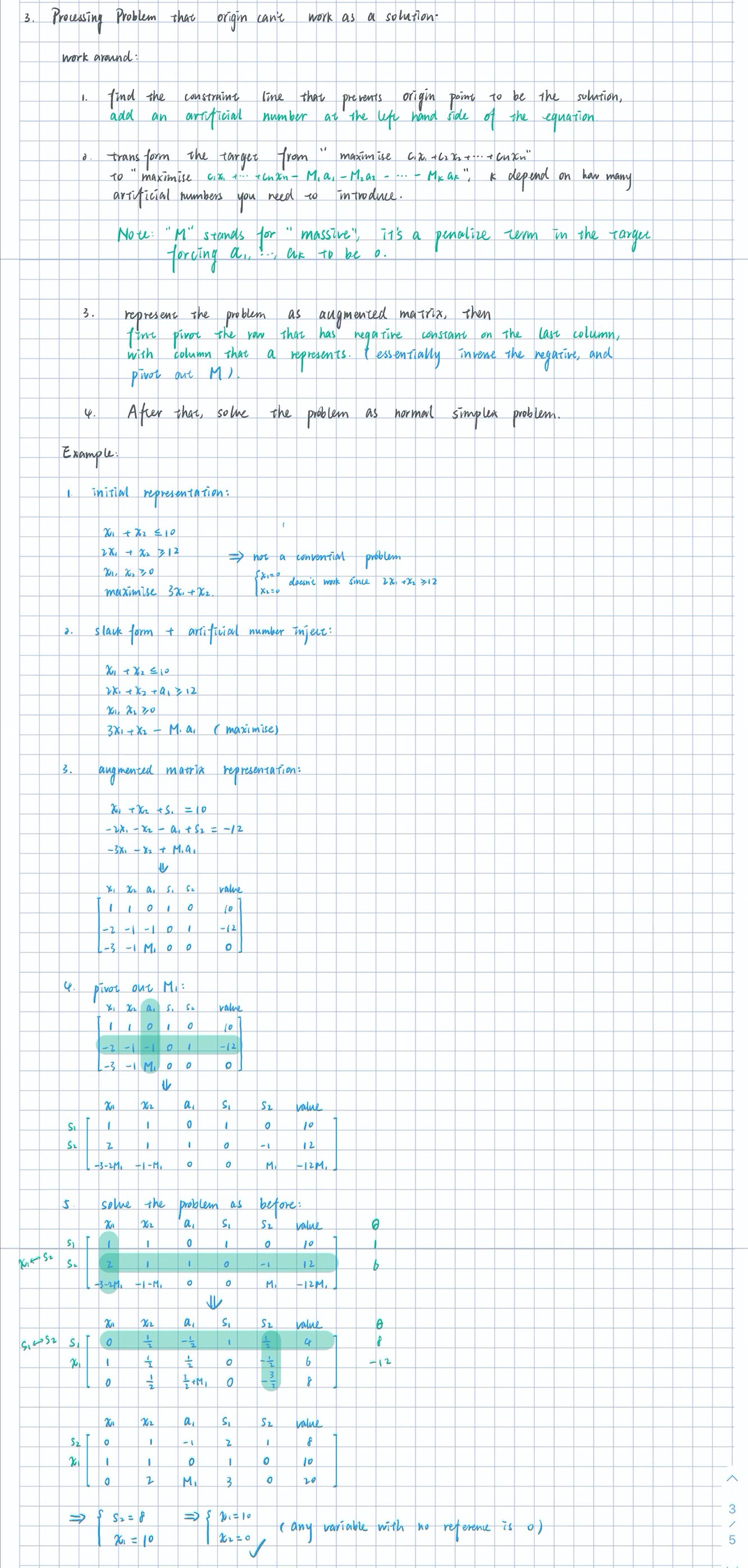## 线性约束问题解空间无界 (Unbounded) 的情形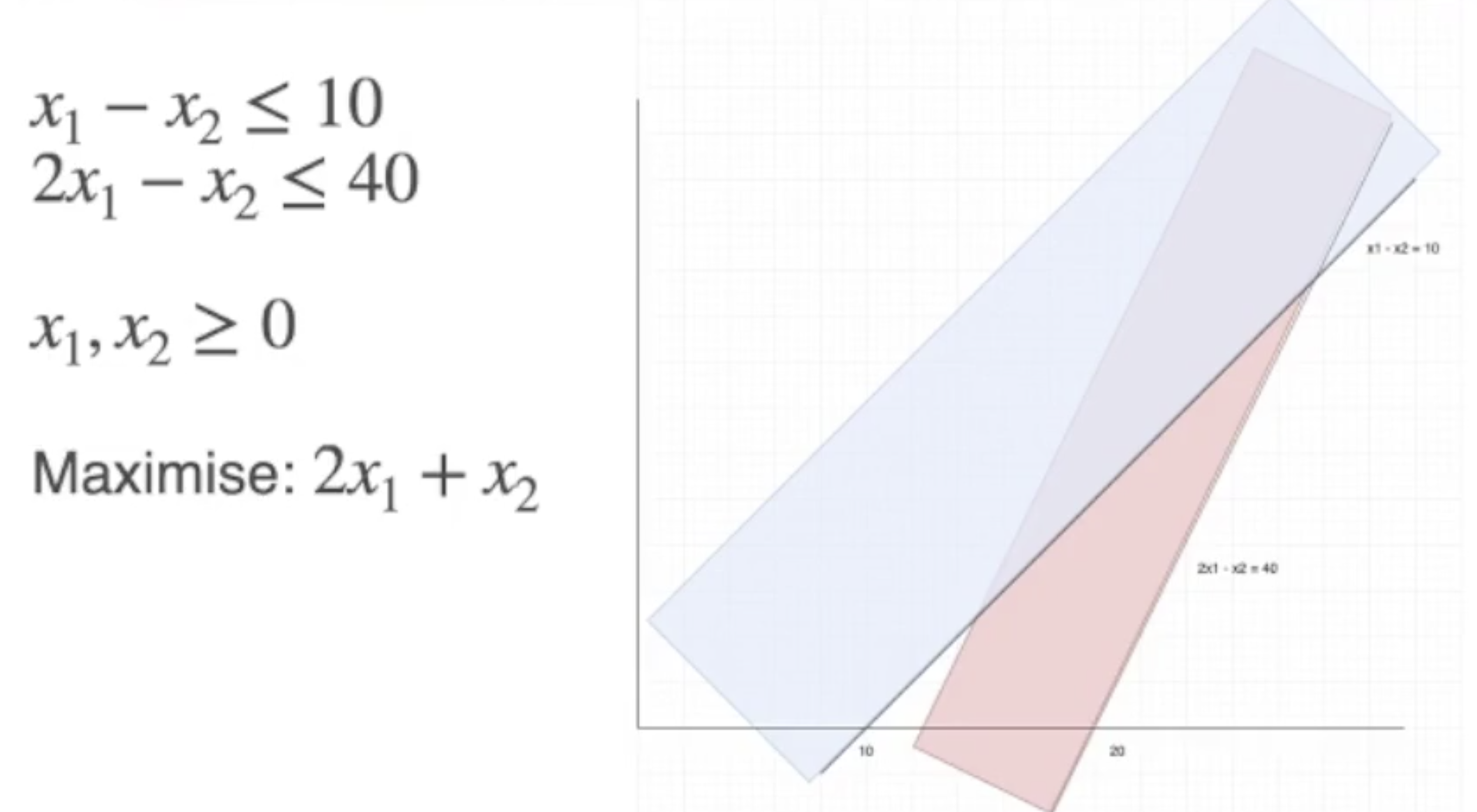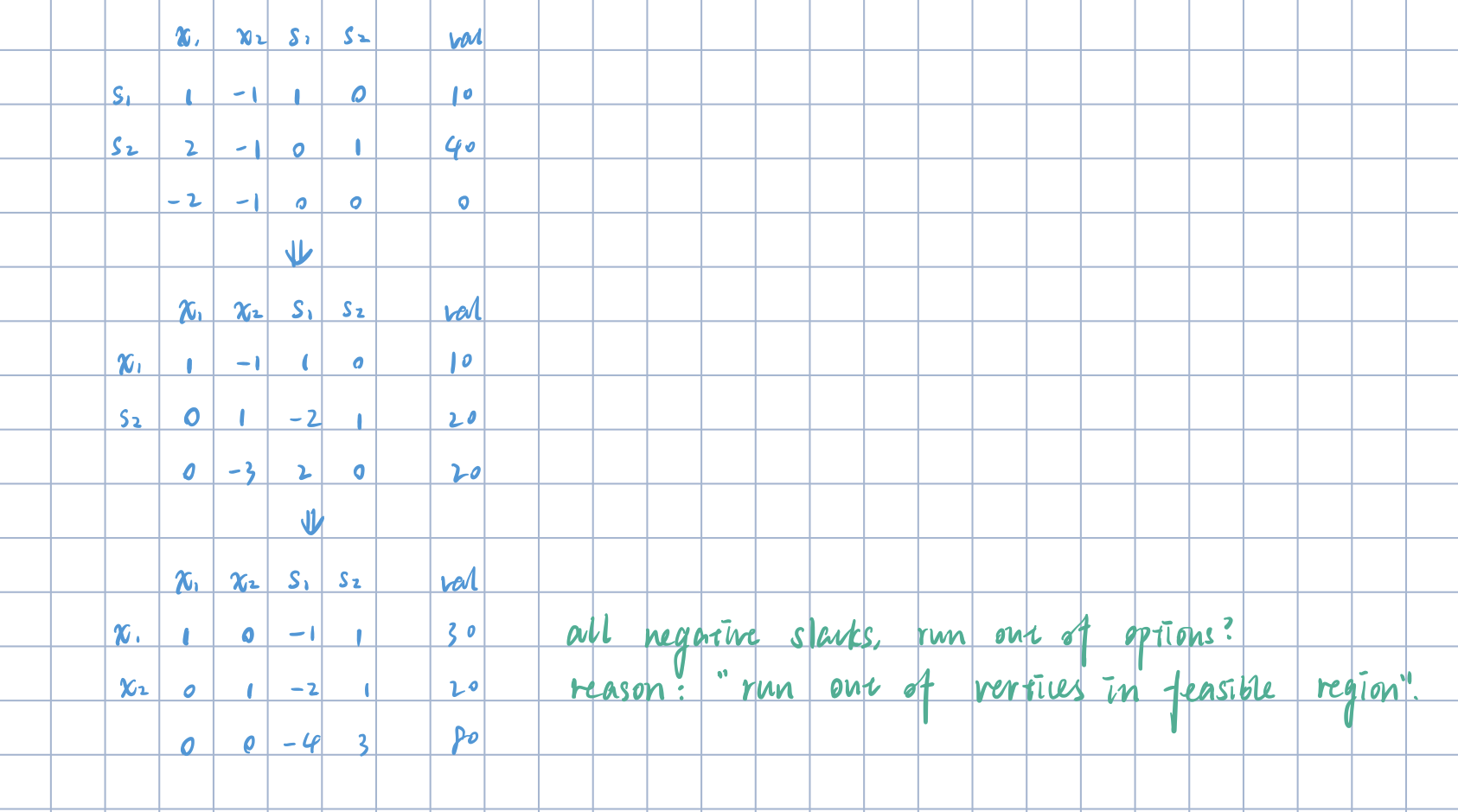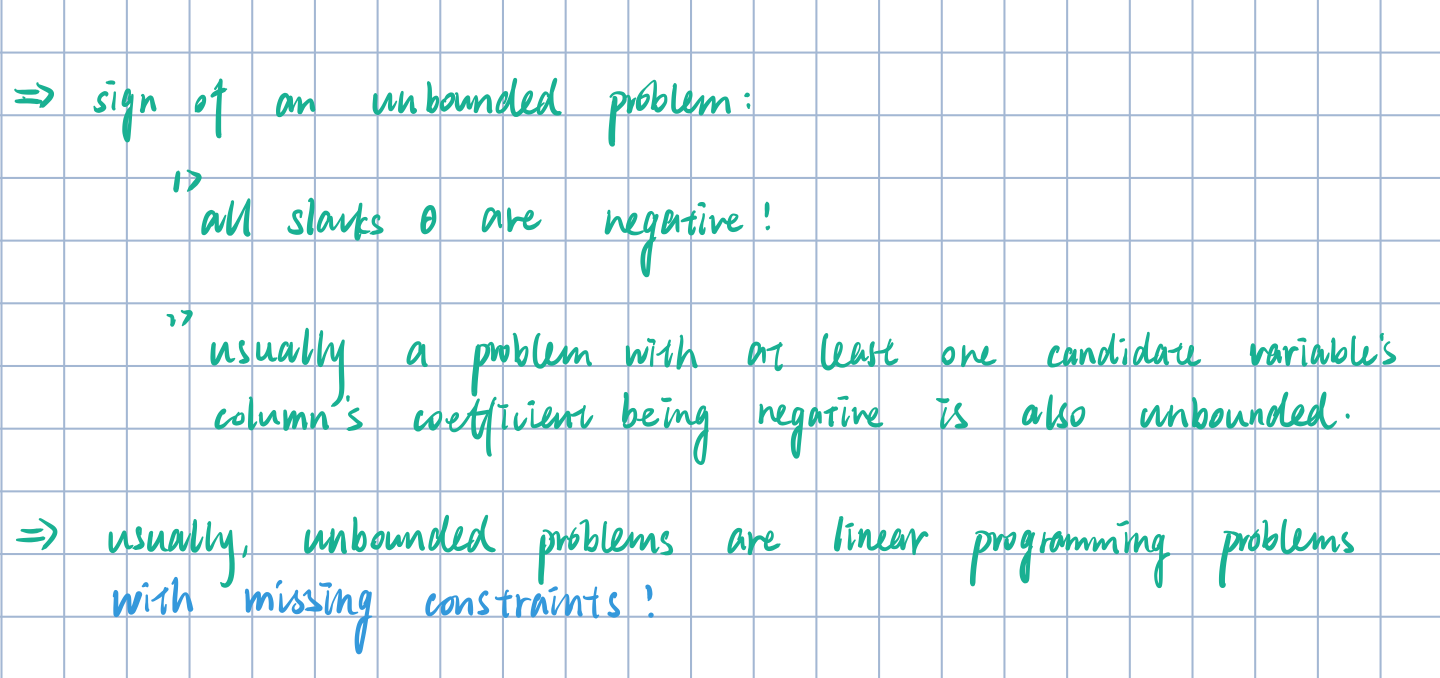## Zero Slack: Degeneracy 问题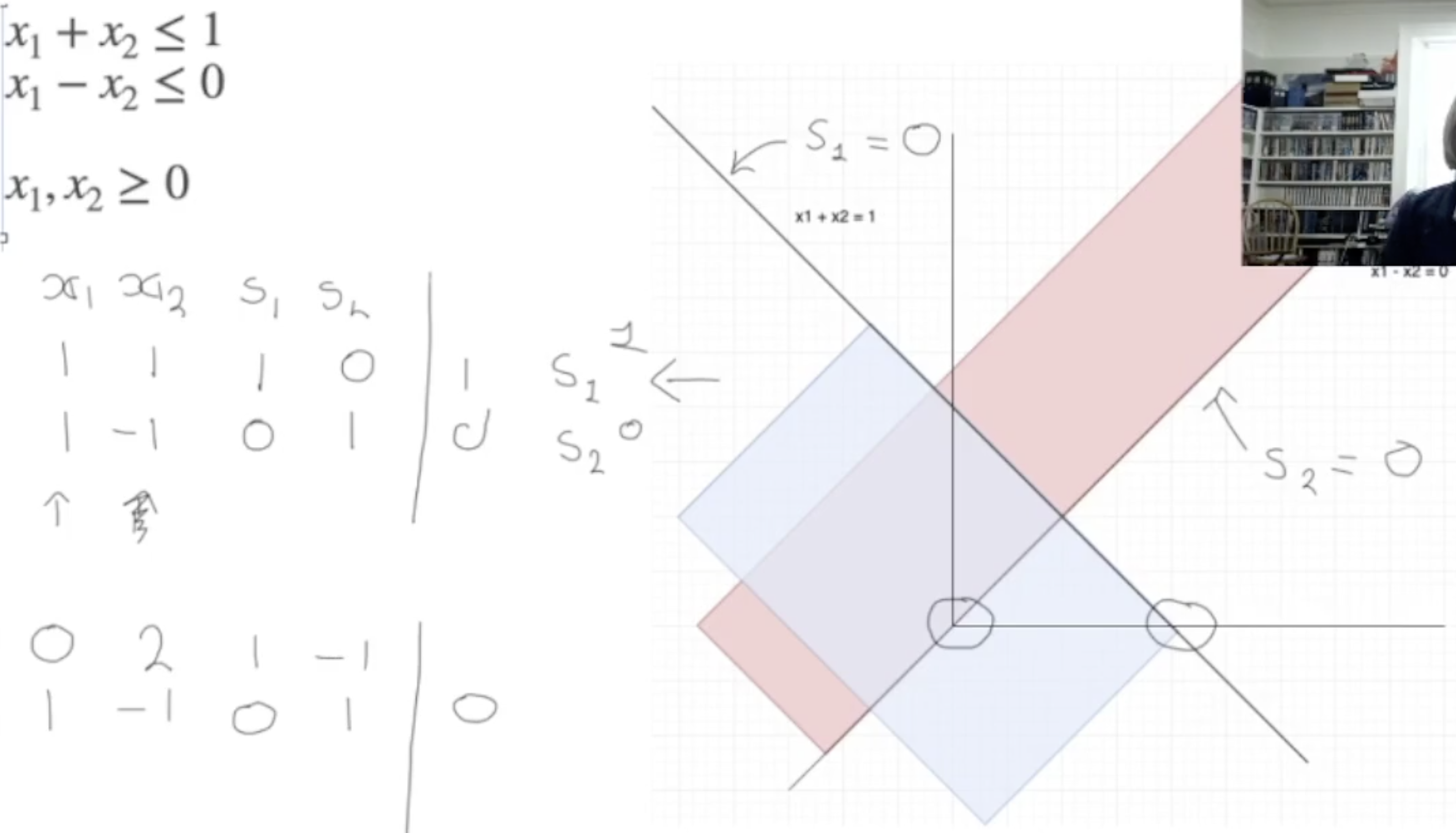” Towards the end of this video I state that, in the case of a choice of leaving variables with zero slack and a positive coefficient in the column for the entering variable you should chose the variable with the largest positive coefficient - this should be smallest positive coefficent (see the reading).”

case1: 若无 zero slack variable with positive coefficient, 选择 $\theta$ 为正数 最小的那个. (注意 $\theta$ 的计算方式: slack variable / coefficient,) case2: 存在一些 zero slack variable with positive coefficient, 此时选择 entering variable 对应列系数 为正且最小的 那一行.

### 補充問題: 單純形法的時間複雜度